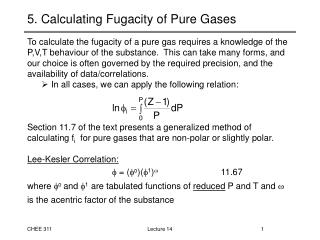DownloadDownload Presentation5. Calculating Fugacity of Pure Gases

# 5. Calculating Fugacity of Pure Gases

Download Presentation## 5. Calculating Fugacity of Pure Gases

- - - - - - - - - - - - - - - - - - - - - - - - - - - E N D - - - - - - - - - - - - - - - - - - - - - - - - - - -
##### Presentation Transcript

1. 5. Calculating Fugacity of Pure Gases • To calculate the fugacity of a pure gas requires a knowledge of the P,V,T behaviour of the substance. This can take many forms, and our choice is often governed by the required precision, and the availability of data/correlations. • In all cases, we can apply the following relation: • Section 11.7 of the text presents a generalized method of calculating fi for pure gases that are non-polar or slightly polar. • Lee-Kesler Correlation: •  = (o)(1) 11.67 • where o and 1 are tabulated functions of reduced P and T and  is the acentric factor of the substance Lecture 14

2. Calculating Fugacity of Pure Gases • Virial Equation: • We have already used another correlation in an example. In cases where the simplest form of the virial equation of state applies, we can calculate fugacity from: • 11.68 • where • 3.65 • 3.66 • See the previously worked out example for a demonstration of this approach. Lecture 14

3. Applicability of Simple Correlations • It is very important to understand under what conditions the simple correlations apply. Lecture 14

4. Pure Component VLE in Terms of Fugacity • Consider a pure component at its vapour pressure: • Phase rule tells us, F=2-2+1 = 1 degree of freedom • Therefore, at a given T, there can only be a single pressure, Psat for which a vapour and a liquid are stable • Along the phase boundary, the chemical potentials are equal • How do the fugacities of the liquid and gas relate? P liquid gas T Lecture 14

5. Pure Component VLE in Terms of Fugacity • For the non-ideal, pure gas we can write: • 11.38a • For a non-ideal liquid, we can define an analogous expression: • 11.38b • At equilibrium, we apply the criterion on the basis of chemical potential to give us: • or • 11.39 • In terms of fugacity coefficients: • 11.41 • All of these equations apply to a pure substance at Pisat Lecture 14

6. Review of Chemical Equilibrium Criteria • We now have several different forms of the criterion for chemical equilibrium. While they stem from the same theory, they differ in practical applicability. • A system at equilibrium has the following properties: • the total Gibbs energy of the system is minimized, meaning that no change in the number of phases or their composition could lower the Gibbs energy further • the chemical potential of each component, i, is the same in every phase within the system in p phases • the fugacity of each component, i, is equal in every phase of the system in p phases Lecture 14

7. Calculating the Fugacity of Pure Liquids • The derivation of the fugacity of a pure liquid at a given T, P is comprised of four steps: • Step 1. Calculate the fugacity of a vapour at Pisat • Step 2. Calculate the change in Gibbs energy between Pisat and the given pressure P using the fundamental equation: • dG = VdP - SdT (constant T) • which after integration yields: • Given that liquids are nearly incompressible (Viliq is not a strong function of P) the integral is easily equated to: • (A) Lecture 14

8. Calculating the Fugacity of Pure Liquids • 3. Using the definitions of fugacity: • we can take the difference: • (B) • 4. Substituting A into B: • or • or • 11.44 Lecture 14

9. Calculating the Fugacity of Pure Liquids • We can now calculate the fugacity of any pure liquid using two equations: • 11.44 • and • 11.35 • The exponential within Equation 11.44 accounts for the change in Gibbs energy as we compress the liquid from Pisat to the specified pressure, P. This is known as the Poynting factor. • This contribution to fugacity is slight at all pressures near Pisat, and is often assumed to be unity. Lecture 14

10. Example Problem* Calculate the change in the chemical potential of pure n-heptane during an isothermal expansion at T=186 oC from its saturation pressure (P1=Psat) to P2=2 bar. Under these conditions, the ideal gas assumption for n-heptane is not valid. Note: the numerical solution to this problem will count for only 5/50. Make good use of your time by simply demonstrating to me the solution procedure (as if you were describing the solution algorithm). Specify the Appendices and Tables that you use. * Midterm Exam Question, Fall 2005 Lecture 14

11. Example Problem Calculate the fugacity of n-pentane at T= 25 oC and P=101 kPa. The saturation pressure of n-pentane at 25 oC is Pisat =67.5kPa. Lecture 14

12. What do we need to know so far (In addition to the bullet items presented in Lecture 3, Slide 15) •How to write the fundamental relations for auxialiary properties, as they apply to open and closed (single, or multi-component) systems • How to calculate S, H, and G changes of mixing for: - Ideal gas mixtures - Perfect solutions • How to express the chemical potential of pure (ideal and non-ideal) compounds (gases and liquids), as well as that of components in ideal mixtures •How to perform P,T-flash calculations (ideal mixtures, only) •How to calculate the fugacity and fugacity coefficients of pure (ideal and non-ideal) substances, existing as gases or liquids Lecture 14